# Potentiometer

You have learned a lot about digital signals and tried many projects with digital devices. Now let’s know something about analog signals. The potentiometer is a typical component to give you analog readings. You'll connect a potentiometer to your circuit and read analog values.

Learning goals

• Learn about analog signals and distinguish them from digital signals.
• Understand the working principle of potentiometers.
• Know how to use the serial monitor.

## 🔸Background​

### What is an analog signal?​

As mentioned before, the signals can be divided into two types: digital signal and analog signal. Unlike the digital signal changing among several values, the analog signal is continuous. Its voltages change smoothly with time, which means there are infinite possible values from minimum to maximum voltage (0 - 3.3V).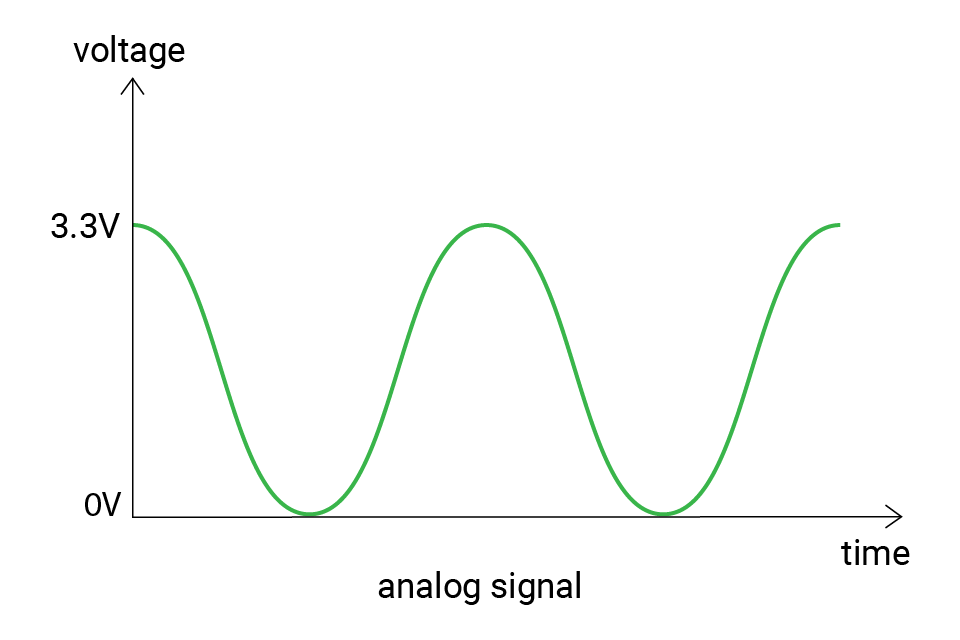But the microcontrollers are digital components, how do they read the analog voltage at a specific time?

Indeed, the microcontrollers cannot directly read analog values. It needs an analog to digital converter (ADC). The ADC converts analog voltages on a pin to corresponding digital values. In this way, the microcontrollers can finally read analog voltages.

The ability to measure analog values depends on the ADC resolution. The resolution tells the count of possible values. For example, if the resolution is 2-bit, there can be 4 possible values: 0, 1, 2, 3. These values are called raw values. And if it's is 3-bit, there are 8 values (0-7).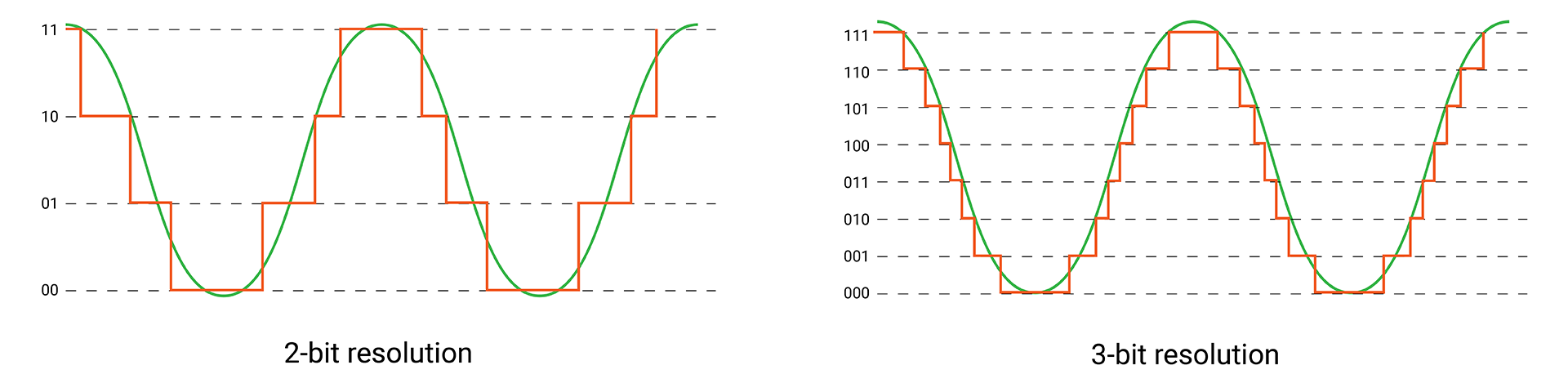You will not look into the details on how ADC works. That's too complex. What you need is the raw values it gives you after reading from an analog signal. These values may be not easy to understand. Then they can be mapped to voltage values between the minimum and maximum voltage.

The SwiftIO Feather board has a built-in 12-bit ADC. So there are 4096 (2^12) analog levels, from 0 to 4095. For example, if the raw value equals 0, the voltage would be 0V; if the raw value equals 4095, the voltage would be 3.3V; and 1365 corresponds to (3.3 / 4095) * 1365 = 1.1V.

Here is the equation for the conversion:

voltage = 3.3 / 4095 * raw value

As you can see, higher resolution means more possible values, so the voltage obtained will be more accurate. Fo instance, as shown in the image above, if you get a raw value of 6 with a 3-bit ADC, the voltage should be around 3.3 / 7 * 6 ≈ 2.8V. But the same voltage might be considered as 3.3V with a 2-bit ADC.

## 🔸New component​

### Potentiometer​

Potentiometer (pot for short, also called knob) is a kind of variable resistor. You can rotate it to change its resistance and thus change the voltage in a circuit. It is commonly used to control the volume on audio devices, as a menu selector knob on home appliances, etc.

The potentiometer has a movable wiper inside it. As you rotate it clockwise or anticlockwise, the wiper moves with it to change the available resistance in the circuit.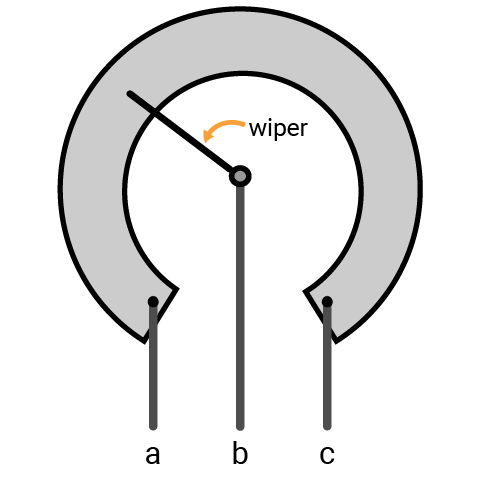Symbols:(international),(US)

As shown in the image above, it has three terminals. The resistance between two ends (a and c) represents the maximum resistance. The middle one is connected to a wiper. As you rotate the knob, the position of the wiper changes and the actual resistance in the circuit changes proportionally.

For example, if you connect the outer pin a to the ground, the other outer pin c to power, and the middle pin b to the input pin, let’s see how it works. When you turn it clockwise, the wiper moves gradually far away from a, the actual resistance between a and b in the circuit increases. And the voltage measured on pin b would increase with it, gradually to 3.3V. If you turn it anticlockwise, the result is the opposite.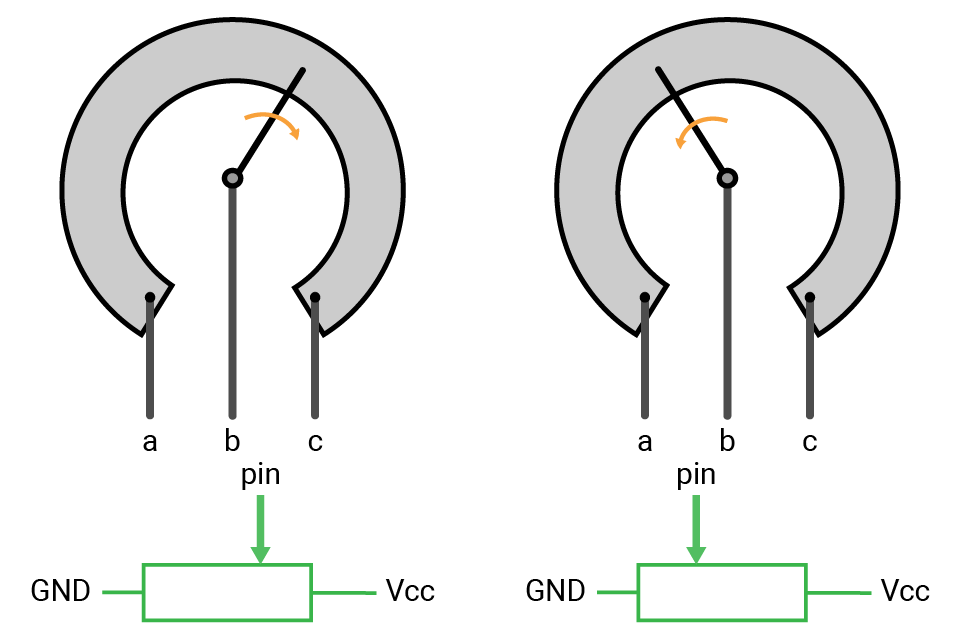## 🔸New concept​

### Voltage divider​

A voltage divider circuit is commonly used to get some smaller voltage levels that are a fraction of the input voltage. Usually, there are resistors connected in series. As the current flows through the resistors, the voltage drops.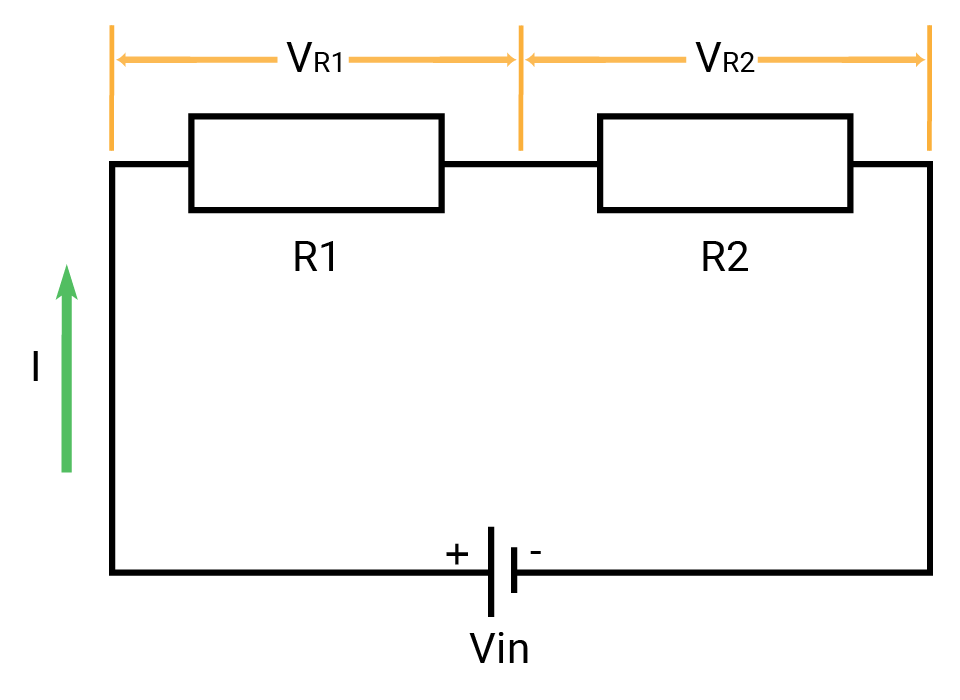The voltage divider calculation is based on the ohm’s law. Here’s the equation: VR1 = Vin * R1/(R1+R2)

• Vin: input voltage
• VR1: voltage drop on R1

Since the two resistors are in series, the current flows through them are the same. The sum of VR1 and VR2 should equal Vin. So the ratio of R1’s resistance to the total resistance equals the ratio of voltage drop to the incoming voltage supply.

The potentiometer is a typical example of it. The whole resistor is cut into two parts by the wiper. As the wiper goes, the ratio of the resistor changes, thus the voltage drop changes accordingly.

## 🔸Circuit - potentiometer module​

There are two potentiometers on your playgrounds. They are connected respectively to A0 and A11.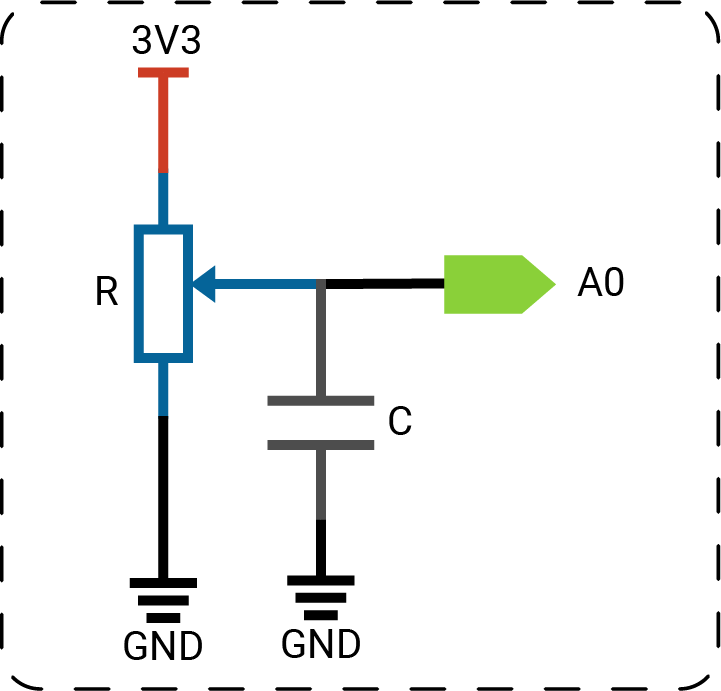note

The circuits above are simplified for your reference.

## 🔸Preparation​

Class

`AnalogIn` - this class allows you to read the analog input.

MethodExplanation
`init(_:)`Initialize an analog pin. The only parameter is the pin id.
`readVoltage()`Read the voltage value on the pin. The return value is a float between 0 and 3.3.
`readPercent()`Read the input value and represent it as a percentage. The return value is between 0 and 1.

Global function

`print(_:)` - print the value out. You can view it on any serial monitor.

## 🔸Projects​

In this project, you will rotate the potentiometer to change the input value. You will view the voltage value on the serial monitor.

After you connect the board to the computer through the serial port, the value is printed on the serial monitor window one by one per second. When you turn the potentiometer, the value changes. It gradually goes to the maximum or minimum value according to the direction you twist.Example code

C01S04Potentiometer.swift
``// Import SwiftIO library to control input and output, and MadBoard to use the pin name.import SwiftIOimport MadBoard@mainpublic struct C01S04Potentiometer {    public static func main() {        // Initialize the analog pin A0 for the potentiometer.        let knob = AnalogIn(Id.A0)        // Read the voltage value and print it out every second.        while true {            let value = knob.readVoltage()            print(value)            sleep(ms: 1000)        }    }}``

Code analysis

``let value = knob.readVoltage()``

Declare a constant to store the returned voltage value.

There are three different methods in `AnalogIn` class to read analog input. The only difference is the forms of the return value: raw value, voltage value, or a percentage. The method `readVoltage()` returns the voltage reading.

``print(value)``

It allows you to see the value on a serial monitor.

It is a useful tool to debug your code if there is something unexpected in your program. You could add it after each statement that changes a value. Then you could infer which step goes wrong according to the printed results.

Serial monitor

A serial monitor is like a link between your board and the computer. You could view the value transmitted between them by using the function `print(_:)`.

If you don't have a preferred serial tool, you could download and install the Serial Studio.

1. Open Serial Studio.

2. Choose the correct port for your board. The port name may be different. If you are not sure which port, you could disconnect your board and see which one disappears.1. Make sure the baud rate is 115200. Choose NL + CR for newline and carriage return. And choose Plain text instead of Hexadecimal for better understanding.1. Click Connect.Once connected, the Connect button will change to Disconnect.### 2. Control buzzer sound output​

You will use a potentiometer to change the sound of the buzzer. The pitch becomes higher when you turn it clockwise and lower when you turn it anticlockwise.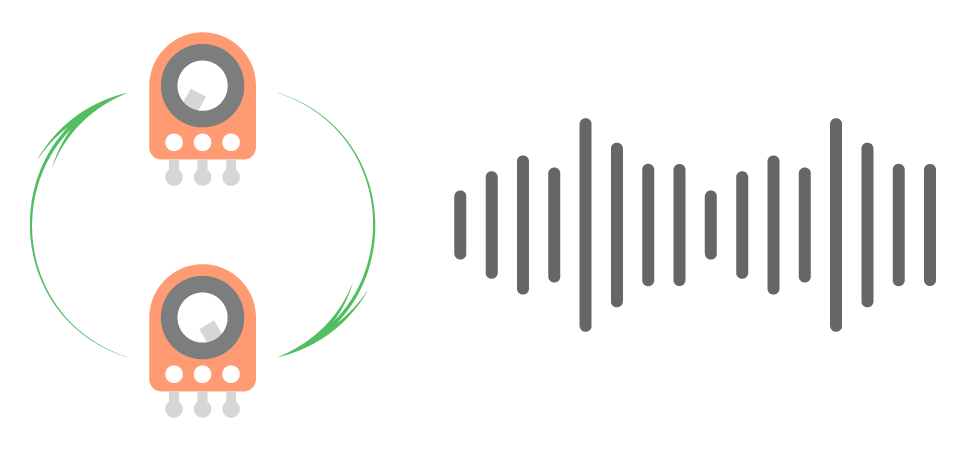Example code

C01S04PotentiometerBuzzer.swift
``// Import the SwiftIO to control input and output and the MadBoard to use the pin name.import SwiftIOimport MadBoard@mainpublic struct C01S04PotentiometerBuzzer {    public static func main() {        // Initialize the analog pin for the potentiometer and PWM pin for the LED.        let knob = AnalogIn(Id.A0)        let buzzer = PWMOut(Id.PWM5A)        // Read the input value in percentage.         // Then calculate the value into the frequency.         // Set the PWM with the frequency and a duty cycle.        while true {            let value = knob.readPercent()            let f = 50 + Int(1000 * value)            buzzer.set(frequency: f, dutycycle: 0.5)            sleep(ms: 20)        }    }}``

Code analysis

``let value = knob.readPercent()``

Here you use another method `readPercent()` to get the input value represented as a percentage.

``let f = 50 + Int(1000 * value)``

The reading values are too small to serve as frequencies. You then map it to bigger values to get frequencies from 50 to 1050.

``buzzer.set(frequency: f, dutycycle: 0.5)``

The PWM pins will output signals with the specified frequencies to drive the buzzer. So when you turn the potentiometer clockwise, the buzzer pitch will increase gradually.

### 3. Change LED brightness​

The potentiometer can also be used to change the brightness of LEDs. In this project, you'll turn the potentiometer to brighten and dim an LED.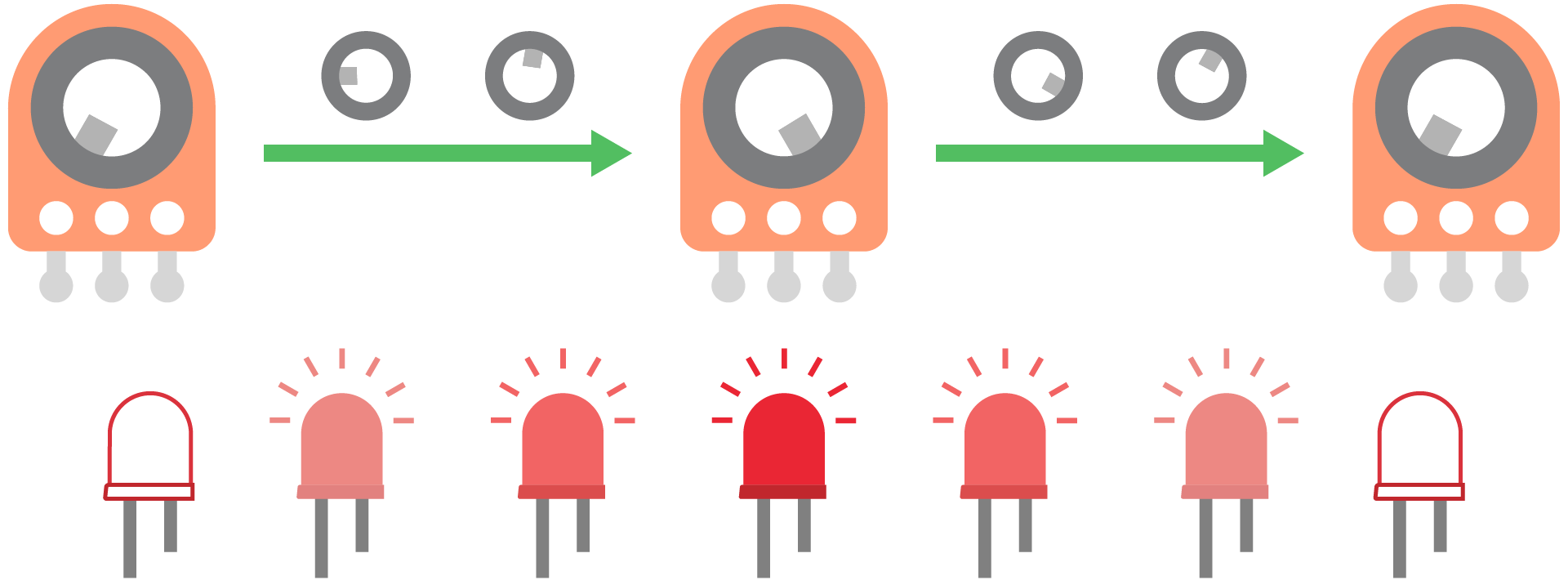Example code

C01S04PotentiometerLED.swift
``// Import SwiftIO library to control input and output, and MadBoard to use the pin name.import SwiftIOimport MadBoard@mainpublic struct C01S04PotentiometerLED {    public static func main() {        // Initialize the analog pin for the potentiometer and PWM pin for the LED.        let knob = AnalogIn(Id.A0)        let led = PWMOut(Id.PWM4A)        // Set the PWM to control the LED.         led.set(frequency: 1000, dutycycle: 0)        // Read the input value.         // The value is represented in percentage, while the duty cycle is also between 0 and 1,         // so you can directly use the reading value to set the PWM.        while true {            let dutycycle = knob.readPercent()            led.setDutycycle(dutycycle)            sleep(ms: 20)        }    }}``